Processing ......FreeComputerBooks.com Links to Free Computer, Mathematics, Technical Books all over the World

Basic Model Theory
🌠 Top Free Computer Networking Books - 100% Free or Open Source!
• Title Basic Model Theory
• Authors Kees Doets
• Publisher: The Center for the Study of Language and Information Publications (13 Jun. 1996)
• Permission: For personal use
• Paperback: 141 pages
• eBook: PDF (141 pages)
• Language: English
• ISBN-10: 1575860481
• ISBN-13: 978-1575860480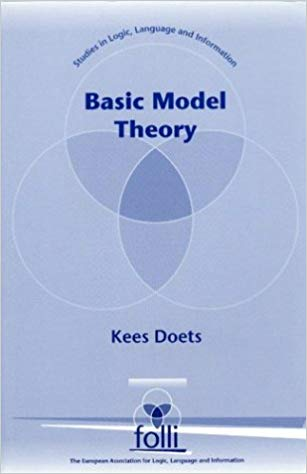Book Description

Model theory investigates the relationships between mathematical structures ('models') on the one hand and formal languages (in which statements about these structures can be formulated) on the other. Example structures are: the natural numbers with the usual arithmetical operations, the structures familiar from algebra, ordered sets, etc. The emphasis is on first-order languages, the model theory of which is best known.

An example result is Lowenheim's theorem (the oldest in the field): a first-order sentence true of some uncountable structure must hold in some countable structure as well. Second-order languages and several of their fragments are dealt with as well.

As the title indicates, this book introduces the reader to what is basic in model theory. A special feature is its use of the Ehrenfeucht game by which the reader is familiarised with the world of models.

• N/A
Reviews, Ratings, and Recommendations: Related Book Categories: Read and Download Links:Similar Books:
•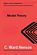Model Theory (C. Ward Henson)

It is an up-to-date textbook of model theory taking the reader from first definitions to Morley's theorem and the elementary parts of stability theory, introduces the model theory of first-order logic, avoiding syntactical issues not too relevant to model theory.

•Model Theory, Algebra, and Geometry (Deirdre Haskell, et al)

Tis book gives the necessary background for understanding both the model theory and the mathematics behind the apps, begins with an introduction to model theory, broadens into three components: pure model theory, geometry, and the model theory of fields.

•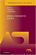Model-Theoretic Logics (Jon Barwise, et al)

This book brings together several directions of work in model theory between the late 1950s and early 1980s. It provides an introduction to the subject as a whole, as well as to the basic theory and examples. Many chapters can be read independently.

•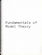Fundamentals of Model Theory (William Weiss, et al)

This book is a concluding discussion focuses on the relationship between proofs and formal derivations, and the role proofs may play as part of a general theory of evidence. It is a primer which will give someone a self contained overview of the subject.

•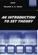An Introduction to Set Theory (William A. R. Weiss)

This book covers the basics: relations, functions, orderings, finite, countable, and uncountable sets, and cardinal and ordinal numbers, gives students sufficient grounding in a rigorous approach to the revolutionary results of set theory.

Book Categories
 :All CategoriesTop Free BooksRecent BooksMiscellaneous BooksComputer EngineeringComputer LanguagesComputer ScienceData Science/DatabasesJava and Java EE (J2EE)Linux and UnixMathematicsMicrosoft and .NETMobile ComputingNetworking and CommunicationsSoftware EngineeringSpecial TopicsWeb Programming
Other Categories